# Correlation between Cyclometric Complexity & Code Coverage while doing White box testing

If you are among those testers (or developers) who have often wondered how cyclometric complexity can practically help me achieve higher code coverage and uncovering max number of bugs by executing minimum no. of unit test then please read on. I have tried to take a very simple example and have focussed on explaining the very basics with data and images only to make sure you can visualize and understand the differences between statement coverage, decision coverage and path coverage and their relationship with cyclometric complexity for once and all.

Pseudocode

if(a>=b)

print  a

else

print b

Do x;

if (a ==0)

print “Zero”

do nothing;

Flow chart of the above pseudocode: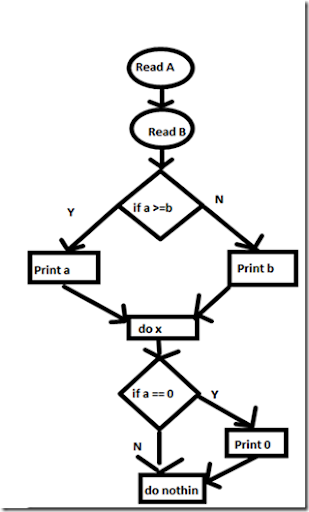Cyclometric Complexity:

= E-N + 2P  (where E is no. of edges, N is the no. of node and P is the no. of connected components in the above graph)

= 10 – 9 + 2 x 1

= 3

Minimum Test Required for 100 % Statement coverage:

Unit Test #1:

Test data: a = 0, b = –1  (True & True)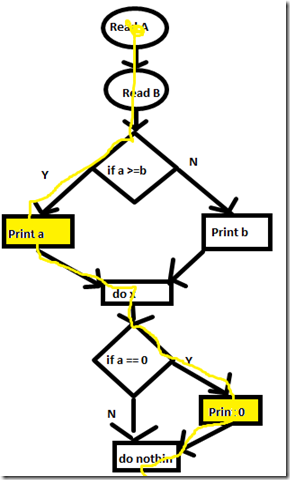Unit Test #2:

Test data = a =0, b = 2 (False & True)

Total  test required for 100 % statement coverage = 2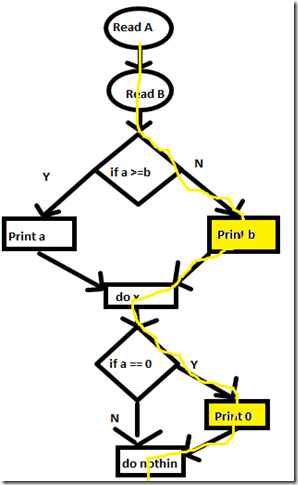With the above two test cases we are able to execute each line of code at least once.

Minimum Test Required for 100 % Branch Coverage or Decision Coverage

Unit Test #1:

Test data: a = 0, b = –1    (True & True)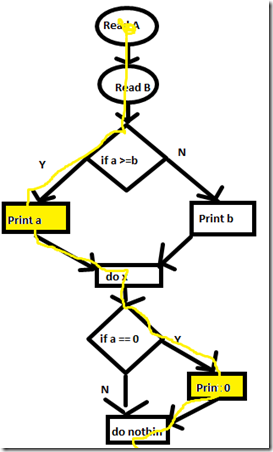Unit Test #2:

Test data: a =1, b = 2  (False & False)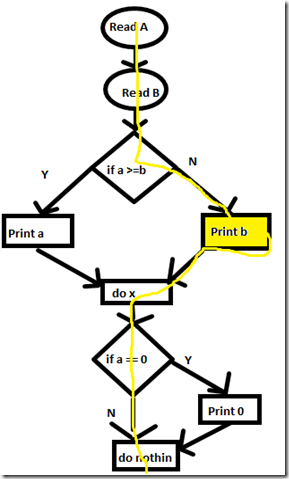Total  test required for 100 % branch coverage = 2

Minimum Test Required for 100 % Path Coverage (Basis Path Coverage)

Unit Test #1:

Test data: a = 0, b = –1  (True & True Input)

< same as scenario #1 of statement coverage>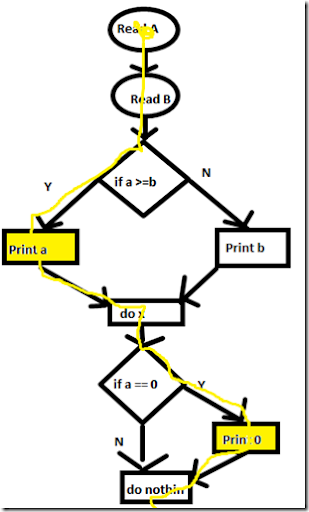Unit Test #2:

Test Data : a = 1, b =0  (True & False Input)

Unit Test #3

Test data:  a =0, b = 2 (False & True Input)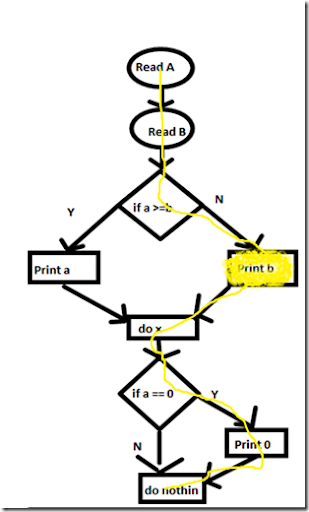Unit Test #4:

Test data:  a =1, b = 2  (False and False Input)

<same as Scenario #2 of branch coverage>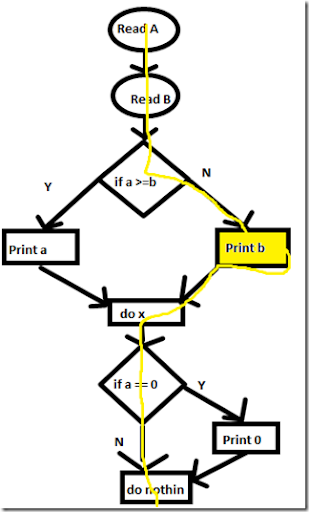Total  test required for 100 % path coverage = 4  (Which is also 2^2 choices where 2 is n decided by first IF-ELSE)

basically what we have done above can also be shown in the form the grid:

 Input\Output First IF O/p of First IF Second IF O/P of Second IF a = 0, b = –1 True Print a True Print 0 a = 1, b =0 True Print a False - a =0, b = 2 False Print b True print a a =1, b = 2 False Print b False -

Universal Formula:

Branch Coverage <= Cyclometric Complexity <= Path Coverage

Replacing our values:

2 <= 3 <= 4 (holds good :))

Now what do we conclude by doing all this:

Does 100 % statement coverage ensures no bugs?

Answer is NO. 100% statement coverage just ensure every statement is executed at least once BUT it doesn’t guarantee that all the conditions are tested for different combination of data and hence it is not the most efficient method

Does 100 % branch coverage ensures no bugs?

Answer is again NO. 100 % branch coverage is certainly better than statement coverage and more efficient but it only ensures that each branch is executed at least once but again it doesn’t cover all the possible combinations  (like two Ifs can be tested with 2 branch test case for TT, FF where as total possible combination will be 4 (TT,TF,FT,FF) which are not getting covered here)

Which is the best way of ensuring 100 % code coverage.

Answer is Path Coverage (a.k.a. basis path testing). This is better than both statement and branch coverage. Though this is practically very impossible to cover all permutations and combinations (For ex when there are 2 IF statements, total paths are 2^2= 4 combination and as no. of Ifs in the program  goes to n, no of possible combination goes to 2^n)

Thats where cyclomertic complexity is used to find out the min test to be executed to achieve path coverage and the max test to be executed for branch coverage.

In above example, if the bug was in a condition when one first IF resulted into True (YES) and Second IF resulted into False (NO) then none statement coverage or branch coverage could find that bug and hence cyclometric complexity = 3 shows that adding one more scenario to branch coverage  as TF or FT (other than TT,FF) will help to uncover that bug so minimum 3 test are required to have high probability of uncovering bugs but max 4 test are required (max possible combinations) to ensure no bugs, which generally become impractical with the growing code containing multiple nested if-else and loops.

Here cyclometric complexity helps unit tester decides at least what are the min no. of test that should be executed to cover the scenarios which have high probability of finding bugs out of total possible scenarios of path coverage (Which is 2^n)

PS: I want to thank Renu (an enthusiastic tester) to bring this topic in our discussions which triggered this interest in me to link all these pieces and try giving an overall perspective to testers by taking this simple example.

Feel free to write your comments to have a discussion or to clarify a point if you disagree.